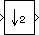# Downsample

Resample input at lower rate by deleting samples

• Library:
• DSP System Toolbox / Signal Operations

DSP System Toolbox HDL Support / Signal Operations

•## Description

The Downsample block decreases the sampling rate of the input by deleting samples. When the block performs frame-based processing, it resamples the data in each column of the Mi-by-N input matrix independently. When the block performs sample-based processing, it treats each element of the input as a separate channel and resamples each channel of the input array across time. The resample rate is K times lower than the input sample rate, where K is the value of the Downsample factor parameter. The Downsample block resamples the input by discarding K–1 consecutive samples following each sample that is output.

This block supports triggered subsystems when you set the Rate options parameter to ```Enforce single-rate processing```.

## Ports

### Input

expand all

Data input whose sampling rate is to be decreased by the block, specified as a column vector or a matrix.

When you set the Input processing parameter to `Elements as channels (sample based)`, the input can be an N-D array.

Data Types: `single` | `double` | `int8` | `int16` | `int32` | `int64` | `uint8` | `uint16` | `uint32` | `uint64` | `Boolean` | `fixed point`
Complex Number Support: Yes

### Output

expand all

Downsampled output with a sampling rate that is 1/K times the input sampling rate, returned as a column vector or a matrix.

Data Types: `single` | `double` | `int8` | `int16` | `int32` | `int64` | `uint8` | `uint16` | `uint32` | `uint64` | `Boolean` | `fixed point`
Complex Number Support: Yes

## Parameters

expand all

The integer factor, K, by which to decrease the input sample rate.

The Sample offset parameter delays the output samples by an integer number of sample periods, D, where 0 ≤ D ≤ (K–1), so that any of the K possible output phases can be selected. For example, when you downsample the sequence 1, 2, 3, ... by a factor of 4, you can select from four phases.

Input SequenceSample Offset, DOutput Sequence (K = 4)

`1,2,3,...`

0

`1,5,9,13,17,21,25,29,...`

`1,2,3,...`

1

`0,2,6,10,14,18,22,26,...`

`1,2,3,...`

2

`0,3,7,11,15,19,23,27,...`

`1,2,3,...`

3

`0,4,8,12,16,20,24,28,...`

The initial zero in each of the latter three output sequences in the table is a result of the default zero Initial conditions parameter setting for this example. See Latency for more information on the Initial conditions parameter.

Specify the method for input processing:

• `Columns as channels (frame based)` –– When you set the Input processing parameter to `Columns as channels (frame based)`, the block treats each of the N input columns as an individual channel containing Mi sequential time samples. The block downsamples each channel independently by discarding K–1 rows of the input matrix following each row that it outputs.

• `Elements as channels (sample based)` –– When you set the Input processing parameter to `Elements as channels (sample based)`, the input can be an N-D array. The Downsample block treats each element of the input as a separate channel, and resamples each channel of the input over time. The block downsamples the input array by discarding K–1 samples following each sample that it passes through to the output. The input and output sizes of the Downsample block are identical.

Specify the method by which the block adjusts the rate at the output to accommodate the reduced number of samples. . You can select one of the following options. The behavior of these options depends on whether the Input processing parameter is set to `Elements as channels (sample based)` (sample-based processing mode) or ```Columns as channels (frame based)``` (frame-based processing mode).

• ```Elements as channels (sample based)```

• ```Enforce single-rate processing```

The block forces the output sample rate to match the input sample rate (Tso = Tsi) by repeating every Kth input sample K times at the output. In this mode, the behavior of the block is similar to the operation of a Sample and Hold block with a repeating trigger event of period KTsi.

• ```Allow multirate processing```

The sample period of the output is K times longer than the input sample period (Tso = KTsi).

• ```Columns as channels (frame based)```

• ```Enforce single rate processing```

The block generates the output at the slower (downsampled) rate using a proportionally smaller frame size than the input. For downsampling by a factor of K, the output frame size is K times smaller than the input frame size (Mo = Mi/K), but the input and output frame rates are equal.

The ex_downsample_ref2 model shows a single-channel input with a frame size of 64 being downsampled by a factor of 4 to a frame size of 16. The input and output frame rates are identical.• ```Allow multirate processing```

The block generates the output at the slower (downsampled) rate by using a proportionally longer frame period at the output port than at the input port. For downsampling by a factor of K, the output frame period is K times longer than the input frame period (Tfo = KTfi), but the input and output frame sizes are equal.

The ex_downsample_ref1 model shows a single-channel input with a frame period of 1 second being downsampled by a factor of 4 to a frame period of 4 seconds. The input and output frame sizes are identical.The initial block value for cases of nonzero latency. You can specify a scalar or an array of the same size as the input.

#### Dependencies

This parameter appears only when you set the Rate options parameter to ```Allow multirate processing```.

## Block Characteristics

 Data Types `Boolean` | `double` | `fixed point` | `integer` | `single` Direct Feedthrough `no` Multidimensional Signals `no` Variable-Size Signals `no` Zero-Crossing Detection `no`

expand all

## Version History

Introduced before R2006a